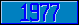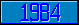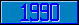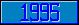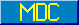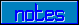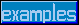## THEN

### M[UMPS] by Example

Approved for inclusion in a future M[UMPS] Language standard.

The command THen stacks the value of special variable \$Test. This value will be "popped" off the stack when execution moves to a different line of code (either through a Goto command, or when the end of the current line is reached).

Assume that the code segment below is executed.

```
For A=1,0 Do

. If A Write !,"TRUE 1" If 0

. Else  Write !,"FALSE 1"

. If A THen  Write !,"TRUE 2" If 0

. Else  Write !,"FALSE 2"

. Quit

```

At the first iteration of the For loop, the value of A is true (1). The first If command will cause the text "TRUE 1" to be written, and the second If command on that line will cause the value of special variable \$Test to be set to 0 (false). As a result, on the next line, the Else command will cause the text "FALSE 1" to be written.

The next If command will cause the text "TRUE 2" to be written, and this time, the THen command will stack the value of \$Test (which is at this point equal to 1). After this, the second If command on that line will cause the value of special variable \$Test to be set to 0 (false). At this point, the end of the line is reached, and the value of \$Test will be popped off the stack, setting it back to 1 (true). As a result, on the next line, the Else command will cause the rest of that line to be ignored.

At the second iteration of the For loop, the value of A is false (0). The first If command will cause the rest of that line to be ignored, and, on the next line, the Else command will cause the text "FALSE 1" to be written.

The next If command will also cause the rest of that line to be ignored, and, on the next line, the Else command will cause the text "FALSE 2" to be written.